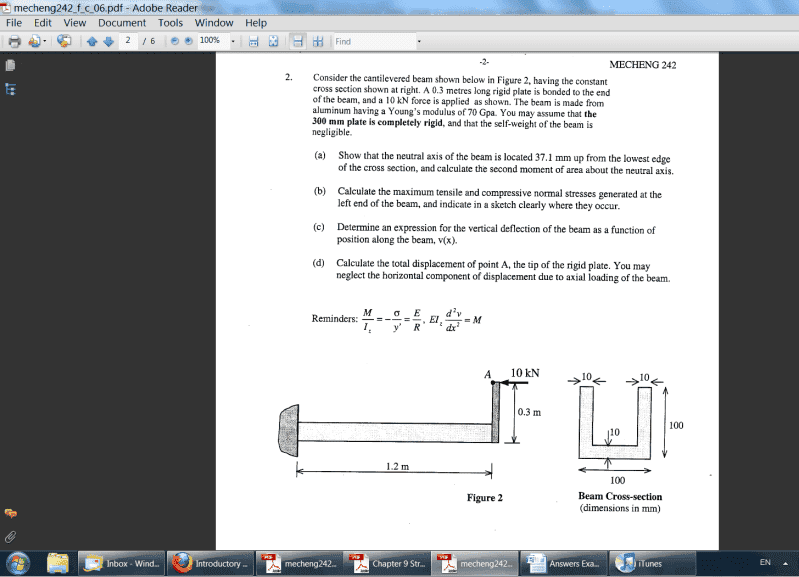# Bending Moment question

## Homework Statement

The problem can be found here:Sorry the picture is so small. I guess you can download it and then zoom in or something. The main question I'm interested in 2b. Calculating the moment.

position of neutral axis: 37.14mm
Second moment of area: 2.83 x 10^(-6)

## Homework Equations

The flexure formula.

## The Attempt at a Solution

My answers for maximum compression : 58.4MPa and maximum tension: 34.5MPa. They're off by about 4Mpa respectively.

PhanthomJay
Homework Helper
Gold Member

Stress tensile=30.93 MPa, Stress compressive=-61.99 MPa.

I can't figure out why I'm wrong.

PhanthomJay
Homework Helper
Gold MemberMe neither,so maybe they're wrong?

I hope not. It was an exam question. I'd imagine they'd be more careful.

Your answers are correct for bending alone, but there is an additional 3.6 MPa for the axial compression. In other words apply the combined stress formula N/A +- M/Z

PhanthomJay